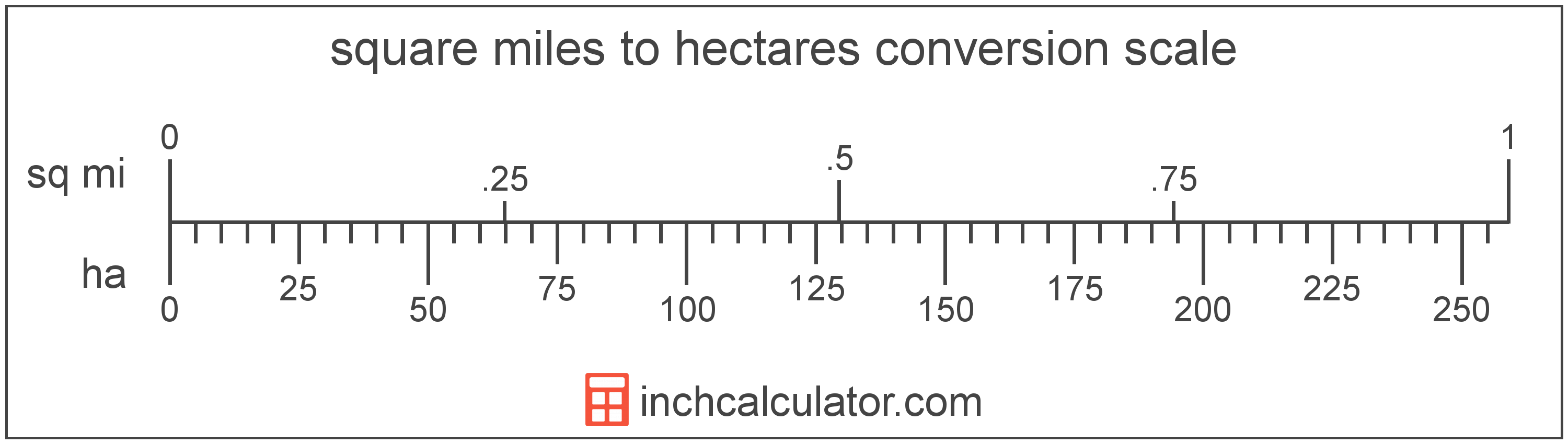# Hectares to Square Miles Converter

Enter the area in hectares below to get the value converted to square miles.

Results in Square Miles:1 ha = 0.003861 sq mi

Do you want to convert square miles to hectares?

## How to Convert Hectares to Square Miles

To convert a measurement in hectares to a measurement in square miles, multiply the area by the following conversion ratio: 0.003861 square miles/hectare.

Since one hectare is equal to 0.003861 square miles, you can use this simple formula to convert:

square miles = hectares × 0.003861

The area in square miles is equal to the area in hectares multiplied by 0.003861.

For example, here's how to convert 500 hectares to square miles using the formula above.
square miles = (500 ha × 0.003861) = 1.930511 sq mi## What is a Hectare?

One hectare is equal to 10,000 square meters, or the area of a square with 100 meter sides.

The hectare is an SI accepted unit for area for use with the metric system. In the metric system, "hecto" is the prefix for 102. Hectares can be abbreviated as ha; for example, 1 hectare can be written as 1 ha.

## What is a Square Mile?

One square mile is equal to the area of a square with sides that are each 1 mile long. One square mile is roughly equal to 2.59 square kilometers or 640 acres.

The square mile is a US customary and imperial unit of area. Square miles can be abbreviated as sq mi, and are also sometimes abbreviated as mi². For example, 1 square mile can be written as 1 sq mi or 1 mi².

## Hectare to Square Mile Conversion Table

Table showing various hectare measurements converted to square miles.
Hectares Square Miles
1 ha 0.003861 sq mi
2 ha 0.007722 sq mi
3 ha 0.011583 sq mi
4 ha 0.015444 sq mi
5 ha 0.019305 sq mi
6 ha 0.023166 sq mi
7 ha 0.027027 sq mi
8 ha 0.030888 sq mi
9 ha 0.034749 sq mi
10 ha 0.03861 sq mi
20 ha 0.07722 sq mi
30 ha 0.115831 sq mi
40 ha 0.154441 sq mi
50 ha 0.193051 sq mi
60 ha 0.231661 sq mi
70 ha 0.270272 sq mi
80 ha 0.308882 sq mi
90 ha 0.347492 sq mi
100 ha 0.386102 sq mi
200 ha 0.772204 sq mi
300 ha 1.1583 sq mi
400 ha 1.5444 sq mi
500 ha 1.9305 sq mi
600 ha 2.3166 sq mi
700 ha 2.7027 sq mi
800 ha 3.0888 sq mi
900 ha 3.4749 sq mi
1,000 ha 3.861 sq mi

## References

1. Cambridge Dictionary, hectare, https://dictionary.cambridge.org/us/dictionary/english/hectare